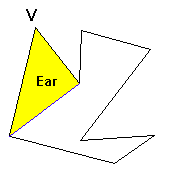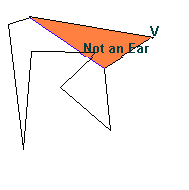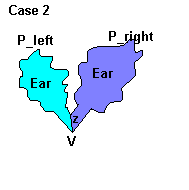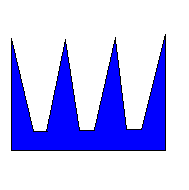Contents

Note : To get the most out of this web organization you are encourraged to read it in the order above. However one of the advantages of html is that you can easily jump back and forth withing the document so feel free to browse.

Introduction : What is the art gallery problem ?

You are the owner of an art gallery and want to place cameras such that the whole gallery will be thief proof.

Where will you place the cameras in a way that the whole gallery is guarded?
How many cameras do you have to buy?
What is the minimum number of cameras required to protect your expensive art collection ?

These are all questions we will answer. The purpose of this page is to familiarize you with the art gallery problem, to demonstrate an algorithm that solves this problem and hopefully to get you interrested in computational geometry problems.

History : The art gallery problem was introduced by Victor Klee in 1973 in a discussion with Vasek Chvatal.
There are many forms of the art gallery problem. We will only look at one of them. Some of the others will be mentionned at the end of this document.

Problem : The gallery will be represented by a simple polygon. Cameras are assumed to have a viewport of 360 degrees. Put otherwise they rotate at infinite speed. Moreover they can see as far as nothing is in their way, i.e until there is a wall. We will ignore the size of the cameras and forget about their vertical positioning. Cameras will be represented by points in the region enclosed by the polygon. The camera sees all points it can be connected to by a segment lying entirely inside the polygon.

Find how many cameras are needed to guard the whole polygon, i.e to view every point inside the polygon.

Where should they be positioned ?

Back to Index

The algorithm : explanation and analysis.

How many cameras would be necessary for a square gallery ?

The answer is evidently one camera. It could be placed anywhere within the polygon. As a matter of fact any convex polygon can be guarded by a single camera. This follows from the definition of a convex pol ygon. The problem becomes interresting when the polygon has a complex shape.

The algorithm we will demonstrate involves dividing the polygon into non-overlapping triangles and placing cameras in each of these triangles. This is called triangulating the polygon. Why would we want to triangulate the polygon? We know on e camera suffices to guard any convex polygon. The triangle is a convex polygon, thus if we triangularize the polygon into say n triangles, we are sure that it can be guarded by n cameras. Of course the polygon may be guarded by less than n cameras (take a square , n=2 but it is guardable by 1 camera), knowing this gives us an upper bound on the number of cameras.

To use this technique we must be sure that any simple polygon can be triangularized. We will prove this is true.

Theorem 1.0: Any simple polygon can be triangulated

This proof uses another theorem about polygons which is called the Two Ears Theorem .This theorem states that any simple polygon must have at least two ears.

Theorem 2.0 : Any simple polygon has two ears.

Of course to be clear we must define what is a polygonal ear. We say that a Polygon P has an ear at vertex V if the triangle formed by V and its two adjacent vertices lies inside P and does not contain a piece of the polygon. To be
precise, there are no vertices of P inside the triangle formed by V and its 2 adjecent vertices. For example :The Two Ears Theorem says that any simple polygon of at least 2 vertices must have two such ears.

Note : This does not hold if P is a triangle. A triangle has only one ear.

Two Ears Theorem : (Proof by induction)

The induction is on the number of vertices of P.

Base Case : (n = 4) We have a quadrilateral. This quadrilateral can be split into 2 triangles which will be the 2 ears of P. The reasonning of the proof is included in the proof for the induction step.

Induction hypothesis: Any simple polygon with less than n vertices has 2 ears. Of course it must have at least 4 vertices.

Induction step: P is a polygon with n vertices. Let V be a concave vertex of P (This eliminates the case where the triangle is outside P). Let v1 and v2 be its two neighboring vertices. Logicaly there are two cases to consider, either there is an ear a t V or there is none.

Case 1: There is an ear at V. Remove this ear from P adding edge (v1,v2) to the remaining set of edges and you obtain another simple polygon. This polygon has n-1 vertices so it must have two ears except if it is a triangle in which case it has one. (from induction hypothesis) It follows that the P had 2 ears.Case 2: There is no ear at V. This means there is at least one vertex in the triangle v1,V,v2. Glide a line parrallel to v1,v2 start from v1 until it reaches the last vertex before V. Call this vertex z. Since there are no points closer to V then z then the segment Vz must lie inside P. Vz divides P into 2 polygons say P_left and P_right where P_left is the part of the polygon that was on the left of Vz with edge Vz added and P_right the other part, also with the new edge Vz. P_left and P_right both have less then n vertices so they both have two ears (Induction hypothesis).

The problem now is to show that this implies P has 2 ears.

It could be that one of P_right and P_left is a triangle. Say it was P_right. Then P_right is an ear of P and P_left must have 2 ears. Surely one of them is not at V or Z. This ear is the second ear of P and P has 2 ears.

It could also be that neither of P_right and P_left is a triangle and in that case by the same reasonning as above, each of P_rigth and P_left must have at least one ear not at either V or Z. These 2 ears are then the 2 ears of P.

End of Proof. (The proof strategy is that of G.H.Meisters)

Back to Index

Proof that any simple polygon can be triangulated: (by construction)

It follows from the 2 ears theorem that we can always find an ear in a simple polygon P. This gives us a method to triangulate a polygon P.

Find an a vertex, say V where there is an ear of P. Remove V and add an edge between its nearest neighbors v1,v2. (note : Edge v1 v2 will be part of the triangulation of P.)

You now have a new Polygon Pnew. Find an ear in Pnew , say at vertex X and do the same as above, storing the neighboring vertices and removing X from Pnew. Do this until you are left with a triangle.

The edges you will have stored are the diagonals which triangulize P.

End of Proof.

Back to Index

We have now shown that any simple polygon can be triangulated and as we have said before, this implies that the polygon can be guarded by n cameras. If you think about it a little bit its obvious that we can often do better than this. We co uld arrang e the cameras so that we only need one to guard 2 triangles in the triangulation. The fact is we can do even better than that. This fact is know as the art gallery theorem.

The Art gallery theorem: Any simple polygon can be guarded by floor (n/3) cameras.

The question now is can we do better than this? The answer is not always. Here is an example where you are forced to use n/3 cameras (12/3 = 4). So the in the worst-case you can guard any simple polygon with floor(n/3) cameras.If you are not convinced that the Art Gallery Theorem is true, here is the proof.

Proof of the Art Gallery Theorem:

Let P be our polygon and T(P) be its triangulation. We will construct a tree from this triangulation such that each node in our tree will represent a distinct triangle in T(P). Edges in our tree will connect nodes whose triangles share a dia gonal. Why is this a tree?

A graph is a tree if :

1. It contains no cycles.
2. It is connected : There is a path connecting any two vertices in the graph.

It is clear that all nodes are connected since all triangles are joined by diagonals. Thus from a node you can reach any other by passing over diagonals of adjacent triangles.

If there were cyles the polygon would have holes. These are not simple polygons since they have more they consist in more then one polygonal chain.

Thus we have a tree. What we will do now is show how to get a 3-coloring of the triangulation of P using this tree.

We say we have a 3-coloring of a polygon when each vertex is assigned one of 3 colors and no adjacent vertices have the same colors. Why do we want to have a 3-coloring? Suppose we have a 3-coloring, then each triangle in our triangulation will have ha ve 3 different colors on its vertices. Thus we can pick one of our three colors and put cameras at the vertices which are of this color. Since all the triangles must contain this color, all triangles will be guarded by a camera and it follows that our wh o le polygon will be guarded.

Here is how to obtain a 3 coloring :

Pick a node in the tree. Color the 3 vertices of its corresponding triangle. Do a depth first traversal of the tree starting at this node. Each time you reach a node it will be because it shares a diagonal with a triangle you have previously colored. T he 2 vertices on this diagonal will therefore be colored and you will be forced to put the unused color on the uncolored vertex of triangle. How do you know that you are free to put this color? Because you are going down a tree and the other adjacent tri a ngles (deeper ones) have not been colored yet.

We have shown we can 3-colour T(P). Once we have the 3-coloring we can compare which color has the least vertices and put cameras on all vertices of this color. Since we have n vertices and 3 colors there can be at most floor(n/3) vertices of the same color.

Note: On the running time of triangulation algorithms.

We have shown that a polygon can be triangulated by showing that any simple polygon has 2 ears. However, this does not in any way garantee us a good running time. The algorithm implied by the proof would do the following :

Triangulate (P)
{
find_diagonal(P);
triangulate(P_left_of_diagonal)
triangulate(P_right_of_diagonal)
}

However finding a diagonal could take as many as n trials. This would lead to an O(n2) time algorithm. As computer scientist we hope to do better that this. The question is , can we?

Yes , the triangulation problem has been arround for a while and there are some very efficient algorithms for polygon triangulation. Some are more complex than others. Some are even unprogrammable. The best algorithms run in O(n) but are very hard to implement.

History of polygon triangulation algorithms.

Back to Index

Complete Algorithm (Pseudo-Code)

Guard-Gallery(P)
{
Triangulation of P = Triangulate(P)
Colored Triangulation = ThreeColor(T(P))
Pick Smallest Color Class
Return Vertices in that Color Class
}

Simple isn't it?

Back to Index

Author : Thierry Dagnino
Web Project : Computational Geometry cs507
Submitted to G.Toussaint
Date : December 7th 1997